﻿ The Block Diagram and Equations of State of the Bond Graph Example

### The Block Diagram and Equations of State of the Bond Graph Example

Darina Hroncová, Alexander Gmiterko, Tomáš Lipták

Journal of Automation and Control

## The Block Diagram and Equations of State of the Bond Graph Example

Darina Hroncová1,, Alexander Gmiterko1, Tomáš Lipták1

1Department of Mechatronics, Faculty of Mechanical Engineering, Technical University of Košice, Letná 9, 042 00 Košice, Slovak republic

### Abstract

The work shows the use the methodology of Bond Graph for modeling electric system of simple RLC circuit. Electrical model is solved by this approach at the level of its physical behavior. In this paper the method of generation of state equations system is discussed. Model of a simple electrical RLC circuit consisting of a resistor, an inductor, and a capacitor is taken. The differential equations describing the dynamics of the system are obtained in terms of the states of the system.

• Darina Hroncová, Alexander Gmiterko, Tomáš Lipták. The Block Diagram and Equations of State of the Bond Graph Example. Journal of Automation and Control. Vol. 3, No. 3, 2015, pp 62-66. http://pubs.sciepub.com/automation/3/3/4
• Hroncová, Darina, Alexander Gmiterko, and Tomáš Lipták. "The Block Diagram and Equations of State of the Bond Graph Example." Journal of Automation and Control 3.3 (2015): 62-66.
• Hroncová, D. , Gmiterko, A. , & Lipták, T. (2015). The Block Diagram and Equations of State of the Bond Graph Example. Journal of Automation and Control, 3(3), 62-66.
• Hroncová, Darina, Alexander Gmiterko, and Tomáš Lipták. "The Block Diagram and Equations of State of the Bond Graph Example." Journal of Automation and Control 3, no. 3 (2015): 62-66.

 Import into BibTeX Import into EndNote Import into RefMan Import into RefWorks

1234
Prev Next

### 1. Introduction

The concept of bond graphs was originated by Paynter. The idea was further developed by Karnopp and Rosenberg in their textbooks, such that it could be used in practice by Thoma, Van Dixhoorn, Breedveld and by others [1, 2, 3, 4].

The language of bond graphs aspires to express general class physical systems through power interactions. The factors of power i.e., effort and flow, have different interpretations in different physical domains. Yet, power can always be used as a generalized coordinate to model coupled systems residing in several energy domains.

A causal bond graph contains all information to derive the set of state equations. The procedure to derive these equations is covered by bond graph software like Enport (, 1974), MS1 (Lorenz, 1997), CAMP (Granda, 1985), and 20-SIM (Broenink, 1990, 1995, 1997, 1999; Broenink and Kleijn, 1999). Therefore, in practice, generation of equation need not be done by hand. However, we discuss the generation of equations to indicate what exactly has to be done.

### 2. Bond Graph of the Electrical System - Description of the Model

The language of bond graphs aspires to express general class physical systems through power interactions. The factors of power i.e., effort and flow, have different interpretations in different physical domains. Yet, power can always be used as a generalized coordinate to model coupled systems residing in several energy domains. To demonstrate the bond graph methodology as an example an electrical model of RLC system is analyzed Figure 1a. An RLC circuit is an electrical circuit consisting of a resistor, an inductor, and a capacitor. The RLC part of the name is due to those letters being the usual electrical symbols for resistance, inductance and capacitance respectively .Download asVeiw figureFigures index
Figure 1. Electrical system: a) electrical model of RLC system; b) reference voltage uref with positive direction; c) efforts (voltages) with unique names u1, u2, u3

### 3. Systematic Procedure to Derive a Bond Graph of the Electrical System

We have discussed the basic bond-graph elements and the bond, so we can transform a domain-dependent ideal-physical model, written in domain-dependent symbols, into a bond graph. For this transformation, there is a systematic procedure, which is presented here. This electrical system contains a voltage source effort SE (SE:uz), a resistor R (R:R), an inductor I (I:L) and a capacitor C (C:1/C). In the step 1 we determine which physical domains exist in the system and identify all basic elements like C (capacitor), I (inductor), R (rezistor), SE (source of the effort), SF (source of the flow), TF (transformer) and GY (gyrator) [6, 7, 8].

This system contains a electrical domain part with the inductance L of the inductor (I:L), the resistance R of the resistor (R:R) and a capacity of the condensator C (C:C). Voltage uz is considered as an effort source (SE: uz).

In the step 2 we indicate in the ideal-physical model per domain a reference source – effort voltage uref (reference voltage with positive direction), Figure 1b. The references are indicated in the ideal physical model: the ideal velocity uref = 0. In the next step 3 we identify all other efforts (voltages) and give them unique names u1, u2, u3, Figure 1c). In step 4 we draw these efforts (electrical: voltages), graphically by 0-junctions in Figure 2a).Download asVeiw figureFigures index
Figure 2. Electrical system (a) voltages are shown as 0-junctions (b) connecting 0-junctions with 1-junctions

We identify in step 5 all effort differences (electrical: voltage = effort) needed to connect the ports of all elements enumerated in step 1 to the junction structure. When checking all ports of the elements found in step 1 linear voltage differences,andare identified: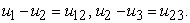(1)

In the step 6 we construct the voltages differences using a 1-junction and draw them as such in the graph Figure 2b). The junction structure is now ready and the elements can be connected.

In the step 7 we connect the port of all elements found at step 1 with the 1-junction of the corresponding efforts or efforts differences in Figure 3a).

We simplify in the step 8 the resulting graph by applying the simplification rules in Figure 3b) and in Figure 4a).Download asVeiw figureFigures index
Figure 3. Electrical system (a) connecting elements R, I, C, SE with 0-junctions (b) simplify the resulting graph by applying the simplification rules

A junction between two bonds can be left out, if the bonds have a through power direction (one bond incoming, the other outgoing). A bond between two the same junctions can be left out, and the junctions can join into one junction. Two separately constructed identical effort or flow differences can join into one effort or flow difference.

In the step 9 we determine the signal direction and causality in Figure 4 b). Causality establishes the cause and effect relationships between the factors of power.Download asVeiw figureFigures index
Figure 4. Simplify the resulting graph by applying the simplification rules (a) the 0-junctions with voltages u2 and u3 (b) determine the causalityDownload asVeiw figureFigures index
Figure 5. Simplify the resulting graph by applying the simplification rules (a) the 0-junctions reduction and causality and (b) the final bond graph with signal direction and causality

In bond graphs, the inputs and the outputs are characterized by the causal stroke. The causal stroke indicates the direction in which the effort signal is directed (by implication, the end of the bond that does not have a causal stroke is the end towards which the flow signal is directed).

There are two ways of describing an element's behavior (e.g. effort in, flow out vs. flow in, effort out) as different causal forms. Note that the two alternative causal forms may, in general, require quite different mathematical operations. The causal form we use, i.e. which variable we select as input and which we select as output, can make a lot of difference. For example, the required mathematical operations may be well defined in one causal form, but not defined at all in the other.

The causal bond graph of this system can be derived, in which the inputs and the outputs are characterized by the causal stroke. This is the starting point, from which we continue toward the differential equations describing the dynamics of the system.

### 4. Expansion to Block Diagrams

The equations are derived in the steps described below.Download asVeiw figureFigures index
Figure 6. The bond graph example – bilateral signal flows between ports and definition of the causal stroke

A causal bond graph in Figure 5b) can be expanded into a block diagram in three steps.

All node symbols in the step 1 are encircled in Figure 7.

Step 2 – All bonds are expanded into bilateral signal flows according to the assigned causality in Figure 7.

In step 3 all constitutive relations of each node are written into block diagram form, according to the assigned causality of each port; one-junction is represented by a signal-node for the flows and a summation for the efforts (Figure 8).

All signals entering a summation resulting from a junction are given a sign corresponding to the half-arrow direction: if, while traveling from causal input to causal output, the bond orientation does not change (this does not exclude an orientation opposite to the signal direction), then a plus sign is added representing a positive contribution to the summation; by contrast if the bond orientation does change, then a minus sign is added representing a negative contribution to the summation. In principle, a complete block diagram is obtained at this point.Download asVeiw figureFigures index
Figure 7. The bond graphs element layout and bond graph with bilateral signals flowDownload asVeiw figureFigures index
Figure 8. The block diagram with the line signal direction

The procedure to obtain a signal flow graph is completely analogous to the above procedure as all operations represented by blocks, including the signs of the summations, are combined as much as possible and then written next to an edge, while all summations become nodes, as signal nodes can be distinguished from signal summation points by observing the signal directions (signal node has only one input, summation has only one output).

#### Table 1. Element in block diagramDownload asPowerPoint Slide

Veiw figure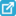View current table in a new windowDownload asVeiw figureFigures index
Figure 9. Bond graph expanded to a block diagram (a) the block diagram with source effort voltage uz

### 5. Generation of the Equations

The two state equations derived from a bond graph diagram (Figure 9) are:(2)(3)

where state variables Φ (t) and Q (t) are magnetic flux and electric charge respectively, , and voltage u23 is: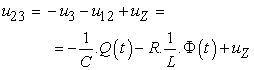(4)

Next equations are: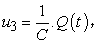(5)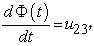(6)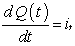(7)(8)(9)

After substitution we get: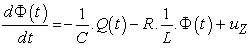(10)(11)

or in matrix form: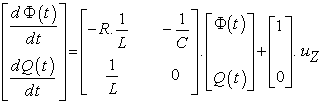(12)

By choosing different state variables i electric current and u3 electric charge, after substitution the state equations are in following form:(13)(14)

and finally:(15)(16)

or in matrix form: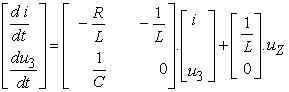(17)

The equation can be solved in Matlab/Simulink .

### 6. State Equation in Matlab/Simulink

Solution of the non-homogenous system of differential equations of a mechanical system with two degrees of freedom is done in Matlab/Simulink using State-Space. The most general state-space representation of a linear system with u(t) inputs, y(t) outputs and n state variables is written in the following form: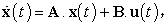(18)(19)

We obtain: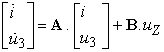(20)(21)

where A, B, C, D are the respective matrices of the mechanical system defined as follows: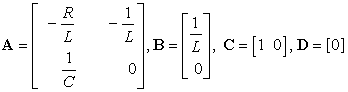(22)

The input u(t) is represented by the source effort voltage uZ :(23)

And the output y(t) is represented by the electric current i:(24)

We determine the matrix and block diagram in Matlab/Simulink shown in Figure 10:Download asVeiw figureFigures index
Figure 10. The block diagram in Simulink

Parameters used in the process of solution of the RLC circuit electrical system (Figure 1a) in Matlab/Simulink  are: R = 5 (Ω), L =0.1 (H), C = 100 (μF) and input voltage uZ = 10 (V).

Output parameters of the electrical current i and voltages uC (uC= u3), uL (uL= u23), (= u12) of the RLC circuit electrical system in Matlab  are shown in Figure 11.Download asVeiw figureFigures index
Figure 11. The results in Matlab uC=uC(t), i=i(t), i=i(uC), uL=uL(t), uR=uR(t), uL=uL(uR)

### 7. Conclusion

Bond graphs represent a convenient tool for physical system analysis. We presented a method to systematically build a bond graph starting from an ideal physical model. Causal analysis gives, besides the computational direction of the signals at the bonds, also information about the correctness of the model. A practical example of an electrical model is presented as the application of this methodology.

The differential equations describing the dynamics of the system in terms of the states of the system were derived from a bond graph diagram of a simple electrical system. The results correspond with equations obtained using traditional method, where the equations for individual components are created first and then the simulation scheme is derived on their basis, although the described method uses the reverse procedure.

### Acknowledgement

This work was supported by grant projects VEGA No. 1/1205/12 and grant projects VEGA No. 1/0937/12.

### References

  Breedveld, P., C., Rosenberg, R., C., Zhou, T., 1991. Bibliography of Bond Graph Theory and Application, Journal of the Franklin Institute, 328(5/6), p.1067-1109.In article View Article  Breedveld, P., C. Modeling and Simulation of Dynamic Systems Using Bond Graphs, http://eolss.net.In article  Broenink, J., F. Introduction to Physical Systems Modelling with Bond Graphs, http://www.ce.utwente.nl.In article  Karnopp, D., C., Margolis, D., L., Rosenberg, R., C., 2005. Modeling and simulation of mechatronic systems. John Willey & Sons, Inc., .In article  Horáček, P., 1999. Systems and models, Nakladatelství ČVUT, Praha, Česko.In article  Gmiterko, A., 2004. Mechatronics, Emilenia, .In article  Gmiterko, A., Šarga, P., Hroncová, D., 2012. Mechatronika I, Technická univerzita Košice, .In article  Hroncová, D., 2012. Studies of compatible software tools for abstract and concrete design of mechatronic systems and linking them for the purpose of logical and physical modeling, Dissertation thesis, .In article  Karban, P., 2006. Výpočty a simulace v programech Matlab a Simulink. Computer Press, , Česko.In article  Virgala, , Frankovský, P., Kenderová, M.: Friction Effect Analysis of a DC Motor. In: American Journal of Mechanical Engineering. 2013. Vol. 1, no. 1 (2013), p. 1-5In article View Article  Vittek, J., 1997. Matlab pre elektrické pohony, Nakladateľstvo Žilinskej univerzity, Žilina, Slovensko, p. 35-36.In article